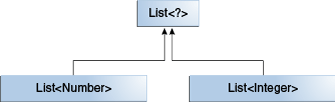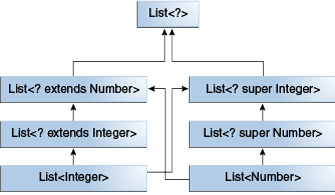Documentation

The Java™ Tutorials
Trail: Learning the Java Language
Lesson: Generics (Updated)
Section: Wildcards

# Wildcards and Subtyping

As described in Generics, Inheritance, and Subtypes, generic classes or interfaces are not related merely because there is a relationship between their types. However, you can use wildcards to create a relationship between generic classes or interfaces.

Given the following two regular (non-generic) classes:

```class A { /* ... */ }
class B extends A { /* ... */ }
```

It would be reasonable to write the following code:

```B b = new B();
A a = b;
```

This example shows that inheritance of regular classes follows this rule of subtyping: class B is a subtype of class A if B extends A. This rule does not apply to generic types:

```List<B> lb = new ArrayList<>();
List<A> la = lb;   // compile-time error
```

Given that Integer is a subtype of Number, what is the relationship between List<Integer> and List<Number>?The common parent is List<?>.

Although Integer is a subtype of Number, List<Integer> is not a subtype of List<Number> and, in fact, these two types are not related. The common parent of List<Number> and List<Integer> is List<?>.

In order to create a relationship between these classes so that the code can access Number's methods through List<Integer>'s elements, use an upper bounded wildcard:

```List<? extends Integer> intList = new ArrayList<>();
List<? extends Number>  numList = intList;  // OK. List<? extends Integer> is a subtype of List<? extends Number>
```

Because Integer is a subtype of Number, and numList is a list of Number objects, a relationship now exists between intList (a list of Integer objects) and numList. The following diagram shows the relationships between several List classes declared with both upper and lower bounded wildcards.A hierarchy of several generic List class declarations.

The Guidelines for Wildcard Use section has more information about the ramifications of using upper and lower bounded wildcards.

Previous page: Lower Bounded Wildcards
Next page: Wildcard Capture and Helper Methods